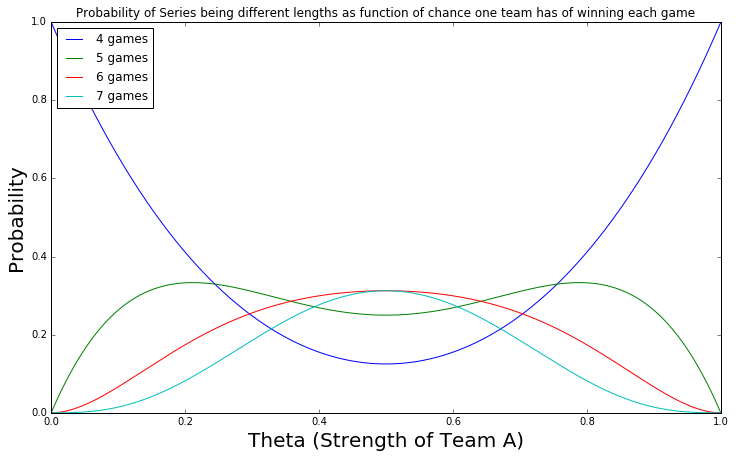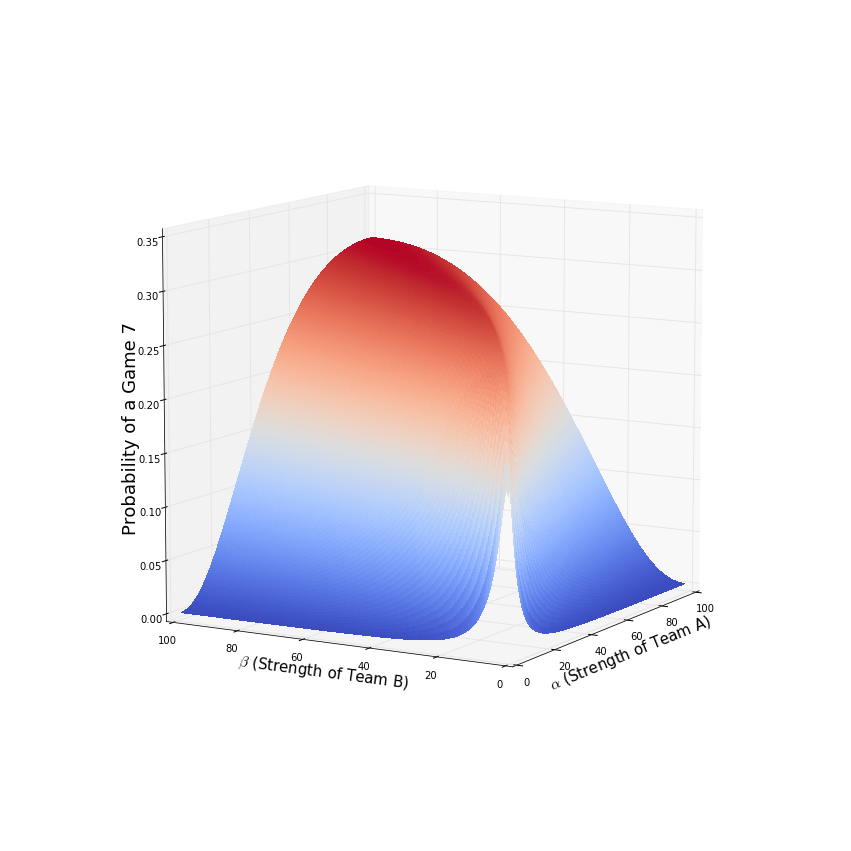# World Series Math

World Series Math

The world series has gone to a 7th game! What are the odds of this happening? Well that depends on how good of a chance each team has of winning each game. There are a couple different approaches we can take - one simple and one a little more complicated.

We can make the simplifying assumption that each game is independent and that the probability of each team winning a game is static. Or we could make it more complicated by updating the probability that a team wins the next game if they've won the previous game. In other words, given the fact that a team wins a game, they will be more likley to win the next. This will involve bayesian updating.

### Part 1 - Strength of Teams Fixed¶

First let's start with the simple approach. Let g, the number of games a series will go, be a discrete random variable that can take on values of 4, 5, 6 or 7. Let $\theta$ be the probability that team A wins. Then,

$$P(g=4 \vert \theta = p) = p^4 + (1-p)^4$$
$$P(g=5 \vert \theta = p) = {4\choose1}*p^4*(1-p) + {4\choose1}*(1-p)^4*p$$
$$P(g=6 \vert \theta = p) = {5\choose2}*p^4*(1-p)^2 + {5\choose2}*(1-p)^4*p^2$$
$$P(g=7 \vert \theta = p) = {6\choose3}*p^3*(1-p)^3$$

There are a bunch of equivelent ways of writing those probabilities. I wrote them here in a way that seemed most elegent, not necessarily the way that is easiest to understand.

Let's do it in Python!

In :
from scipy.misc import comb

def pmf_games(p):
p_4 = p**4 + (1-p)**4
p_5 = comb(4,1) * (p**4 * (1-p) + (1-p)**4 * p)
p_6 = comb(5,2) * (p**4 * (1-p)**2 + (1-p)**4 * p**2)
p_7 = comb(6,3) * (p**3 * (1-p)**3)
return (p_4,p_5,p_6,p_7)

In :
pmf_games(.5)

Out:
(0.125, 0.25, 0.3125, 0.3125)

Ok, given the teams are equally likley to win any game, the probability that the series goes to 7 games is .3125. Before we plot, let's do a quick sanity check:

In :
import numpy as np
np.allclose([sum(pmf_games(theta)) for theta in np.linspace(0,1,100)],*100)

Out:
True

Phew, now let's plot!

In :
import matplotlib.pyplot as plt
%matplotlib inline

plt.figure(figsize=(12,7))
plt.plot(np.linspace(0,1,1000),[pmf_games(x) for x in np.linspace(0,1,1000)],label='4 games');
plt.plot(np.linspace(0,1,1000),[pmf_games(x) for x in np.linspace(0,1,1000)],label='5 games');
plt.plot(np.linspace(0,1,1000),[pmf_games(x) for x in np.linspace(0,1,1000)],label='6 games');
plt.plot(np.linspace(0,1,1000),[pmf_games(x) for x in np.linspace(0,1,1000)],label='7 games');
plt.legend(loc=2);
plt.xlim(0,1);
plt.xlabel('Theta (Strength of Team A)',fontsize=20)
plt.ylabel('Probability',fontsize=20)
plt.title('Probability of Series being different lengths as function of chance one team has of winning each game');Cool looking plot!

### Part 2 - Strength of Teams Updating¶

Now let's do away with the simplifying assumption and say that the probaility of a team winning the next game increases if they win their last one. We come in with some prior belief of how good the teams are, then we update the belief as we see more data. In other words, we take into account the possibility of momentum in the series.

Instead of being static, now we will take $\theta$ to be a random variable with a Beta distribution described by some choice for the hyperparameters $\alpha$ and $\beta$. We could be silly and let $\alpha = \beta = 1$ meaning that we have no idea what $\theta$ is just "let the data decide". For fun, let's calculate the chances of the series being different lengths for a range of choices of hyperparameters. Maybe we can make some more interesting looking plots.

In makes intuitive sense that any amount of 'momentum' would make the series be shorter given the same expectation of $\theta$. The less informative the prior, the more momentum there should be, and the shorter the chance of a long series.

Let's see if the numbers bear this out. Remember $\theta$ is the belief we have in team A. And it is a Beta-distributed random variable with some prior choice of $\alpha$ and $\beta$. Let's start with the probability the series is a sweep:

$$P(g=4 \vert \alpha,\beta) = (\frac{\alpha}{\alpha+\beta}) (\frac{\alpha+1}{\alpha+\beta+1}) (\frac{\alpha+2}{\alpha+\beta+2}) (\frac{\alpha+3}{\alpha+\beta+3}) + (\frac{\beta}{\alpha+\beta}) (\frac{\beta+1}{\alpha+\beta+1}) (\frac{\beta+2}{\alpha+\beta+2}) (\frac{\beta+3}{\alpha+\beta+3})$$

Ok, that was not that bad. The next one, however, will not be as easy to write out. The problem results from the lack of exchangability between the different sequences of possible results. With a static estimate of $\theta$, the probability of this sequence of winners - ABAAA - is the same as this sequence of winners - AABAA. However, when we update our estimate of $\theta$ after each result, the first sequence is actually more probable than the second.

We actually need to calculate all of there sequences explicitly. I won't write it out but I will do the calculation.

Here are the sequences we need to consider for the series ending in 5 games. The trick here is realizing that the series must end with a win from the winning team.

In :
import itertools
A_wins = set(itertools.permutations('AAAB'))
A_wins = map(lambda x: list(x)+['A'],A_wins)
B_wins = set(itertools.permutations('BBBA'))
B_wins = map(lambda x: list(x)+['B'],B_wins)

In :
A_wins + B_wins

Out:
[['A', 'A', 'A', 'B', 'A'],
['A', 'B', 'A', 'A', 'A'],
['A', 'A', 'B', 'A', 'A'],
['B', 'A', 'A', 'A', 'A'],
['A', 'B', 'B', 'B', 'B'],
['B', 'B', 'A', 'B', 'B'],
['B', 'A', 'B', 'B', 'B'],
['B', 'B', 'B', 'A', 'B']]

I'll write a class to enclose the whole calculation.

In :
class Process():

def __init__(self,alpha,beta):
## save two copys of parameters, one static one changing
self.prior_alpha,self.alpha = float(alpha),float(alpha)
self.prior_beta,self.beta = float(beta),float(beta)

def get_seq(self,g,winner):
if winner=='A':
seq = 'A' * 3 + 'B' * (g-4)
perms = set(itertools.permutations(seq))
seqs = map(lambda x: list(x) + ['A'],perms)
else:
seq = 'B' * 3 + 'A' * (g-4)
perms = set(itertools.permutations(seq))
seqs = map(lambda x: list(x) + ['B'],perms)
return seqs

def calculate(self):
p_4,p_5,p_6,p_7 = 0,0,0,0

seq_4 = self.get_seq(4,'A') + self.get_seq(4,'B')
seq_5 = self.get_seq(5,'A') + self.get_seq(5,'B')
seq_6 = self.get_seq(6,'A') + self.get_seq(6,'B')
seq_7 = self.get_seq(7,'A') + self.get_seq(7,'B')

## all possibilities for a series of length 4
for seq in seq_4:
self.alpha,self.beta = self.prior_alpha,self.prior_beta
p_4 += self.get_prob(seq)

## all possibilities for a series of length 5
for seq in seq_5:
self.alpha,self.beta = self.prior_alpha,self.prior_beta
p_5 += self.get_prob(seq)

## all possibilities for a series of length 6
for seq in seq_6:
self.alpha,self.beta = self.prior_alpha,self.prior_beta
p_6 += self.get_prob(seq)

## all possibilities for a series of length 7
for seq in seq_7:
self.alpha,self.beta = self.prior_alpha,self.prior_beta
p_7 += self.get_prob(seq)

return (p_4,p_5,p_6,p_7)

def get_prob(self,seq):
p = 1
for winner in seq:
if winner=='A':
p *= self.alpha / (self.alpha + self.beta)
self.A_won()
else:
p *= self.beta / (self.alpha + self.beta)
self.B_won()
return p

def A_won(self):
self.alpha += 1

def B_won(self):
self.beta += 1


Let's see how it works! First, quick sanity check:

In :
for alpha,beta in zip(np.linspace(1,100,100),np.linspace(1,100,100)):
proc = Process(alpha,beta)
assert np.allclose([sum(proc.calculate())],)


Ok, cool. Now let's see what we calculate with a completely uninformative prior - meaning before the series $\theta$ has a uniform distribution on (0,1).

In :
proc = Process(1,1)
proc.calculate()

Out:
(0.4, 0.2666666666666666, 0.19047619047619038, 0.14285714285714282)

Ok, so if we admit no prior knowledge about the strength of the teams, we calculate that the series has only a 14% chance of going to seven games instead of the 31% we got before. With this uninformative prior, even the small amount of data from previous games overwhelms the prior and therefore we calculate that the series will end faster.

If we maintain the prior that the strength of the teams is equal, meaning $\alpha = \beta$, but we increase our certainty in this belief, we should recover the estimate that we made when we took $\theta$ to be static. Let's see if this is the case..

In :
proc = Process(5,5)
print proc.calculate()

(0.1958041958041958, 0.27972027972027963, 0.27972027972027974, 0.24475524475524477)

In :
proc = Process(50,50)
print proc.calculate()

(0.13246178986830723, 0.2547342112852062, 0.3093201137034647, 0.3034838851430219)

In :
proc = Process(500,500)
print proc.calculate()

(0.12574962425411085, 0.2504972594703403, 0.3121868830712449, 0.3115662332043041)


This is indeed the case. In the limit, the probabilties will converge to the ones previously arrived at. But that's no fun! The point of this Bayesian framework is the beauty of the middle ground. Just like it is silly to have a completely uninformative prior, it is also silly to have complete certainty in one's beliefs with no willingness to allow data alter them.

In a real application, the prior could be chosen in a variety of manners - using some sort of empirical data or some combination of data and expertise.

I am not that big of a baseball fan. I am more of a math fan. So instead of arguing about the proper priors for this world series or ones in the past, I'll end this post by making a 3D plot. We will plot $\alpha$ vs. $\beta$ vs. $P(g=7)$.

In :
from mpl_toolkits.mplot3d import Axes3D
from matplotlib import cm

In :
## Shoutout numpy for being awesome
@np.vectorize
def f(alpha,beta):
proc = Process(alpha,beta)
return proc.calculate()

In :
X = np.arange(1,100,.5)
Y = np.arange(1,100,.5)
X, Y = np.meshgrid(X, Y)
Z = f(X,Y)


Ok, we are ready to plot.

In :
fig = plt.figure(figsize=(15,15))
ax.set_xlabel(r'$\alpha$ (Strength of Team A)',fontsize=15)
ax.set_ylabel(r'$\beta$ (Strength of Team B)',fontsize=15)